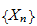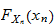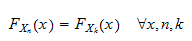StatLect

IID sequence

The acronym IID stands for "Independent and Identically Distributed".

A sequence of random variables (or random vectors) is IID if and only if the following two conditions are satisfied:

1. the terms of the sequence are mutually independent;

2. they all have the same probability distribution.Definition

A formal definition follows.

Definition Letbe a sequence of random vectors. Letbe the joint distribution function of a generic term of the sequence. We say thatis an IID sequence if and only ifand any subset of terms of the sequence is a set of mutually independent random vectors.

Of course, the definition applies also to the special case in which the terms of the sequenceare uni-dimensional, that is, they are random variables.

Usage in asymptotic theory

The requirement that a sequence of random variables be IID is often found in asymptotic theory, for example to derive some versions of the Law of Large Numbers and of the Central Limit Theorem.

However, the requirement that a sequence be IID is very strong and is often replaced by milder conditions such as covariance stationarity or mixing.

Usage in maximum likelihood estimation

The IID assumption is frequently made also in maximum likelihood estimation, where it helps to write down the joint density of the observed sample. In fact, when the observations in a sample are IID:

If want to learn how the IID assumption is used in maximum likelihood (ML) estimation, you could read the following lectures:

More details

More details about IID sequences are presented in the lecture entitled Sequences of random variables.